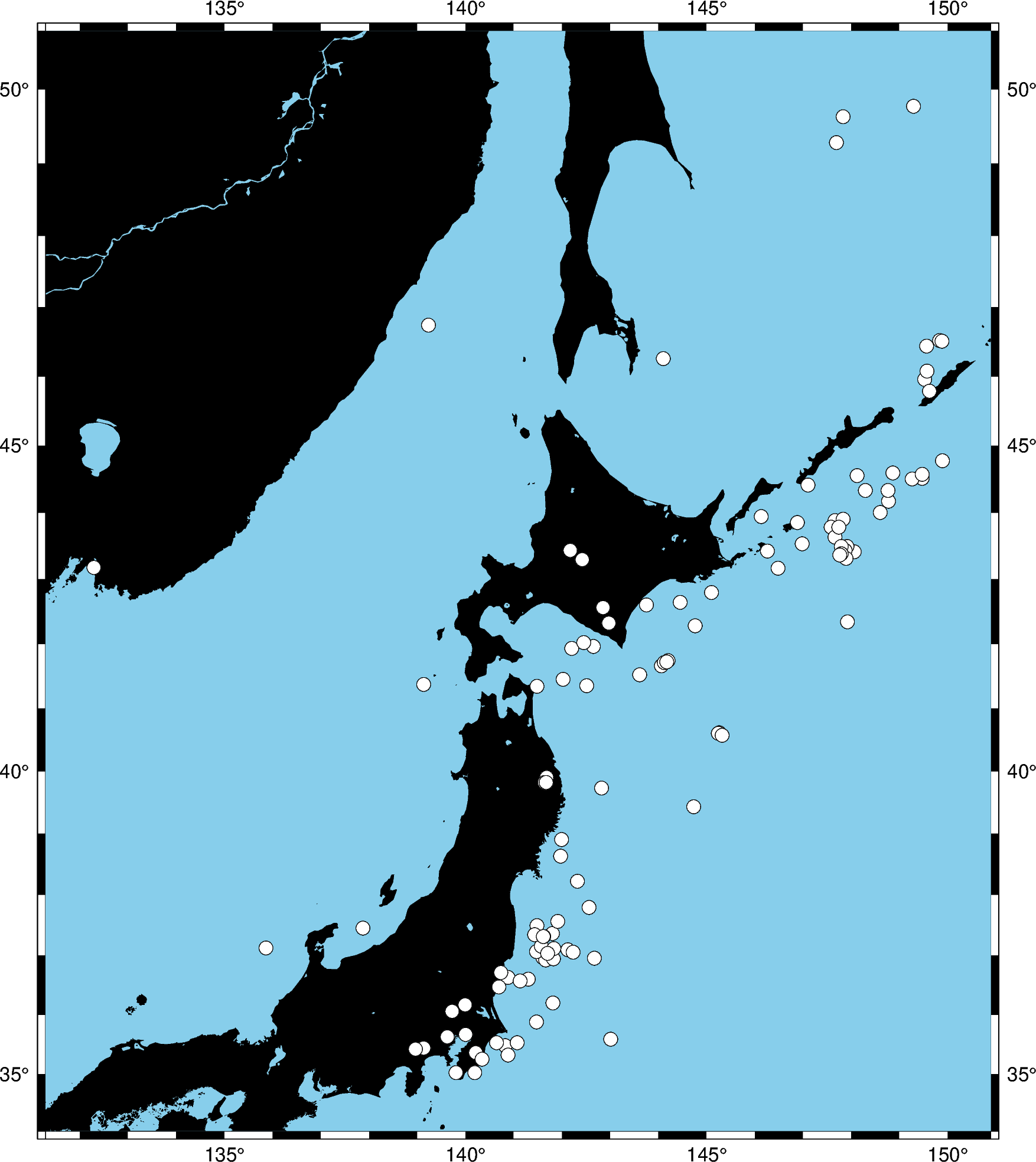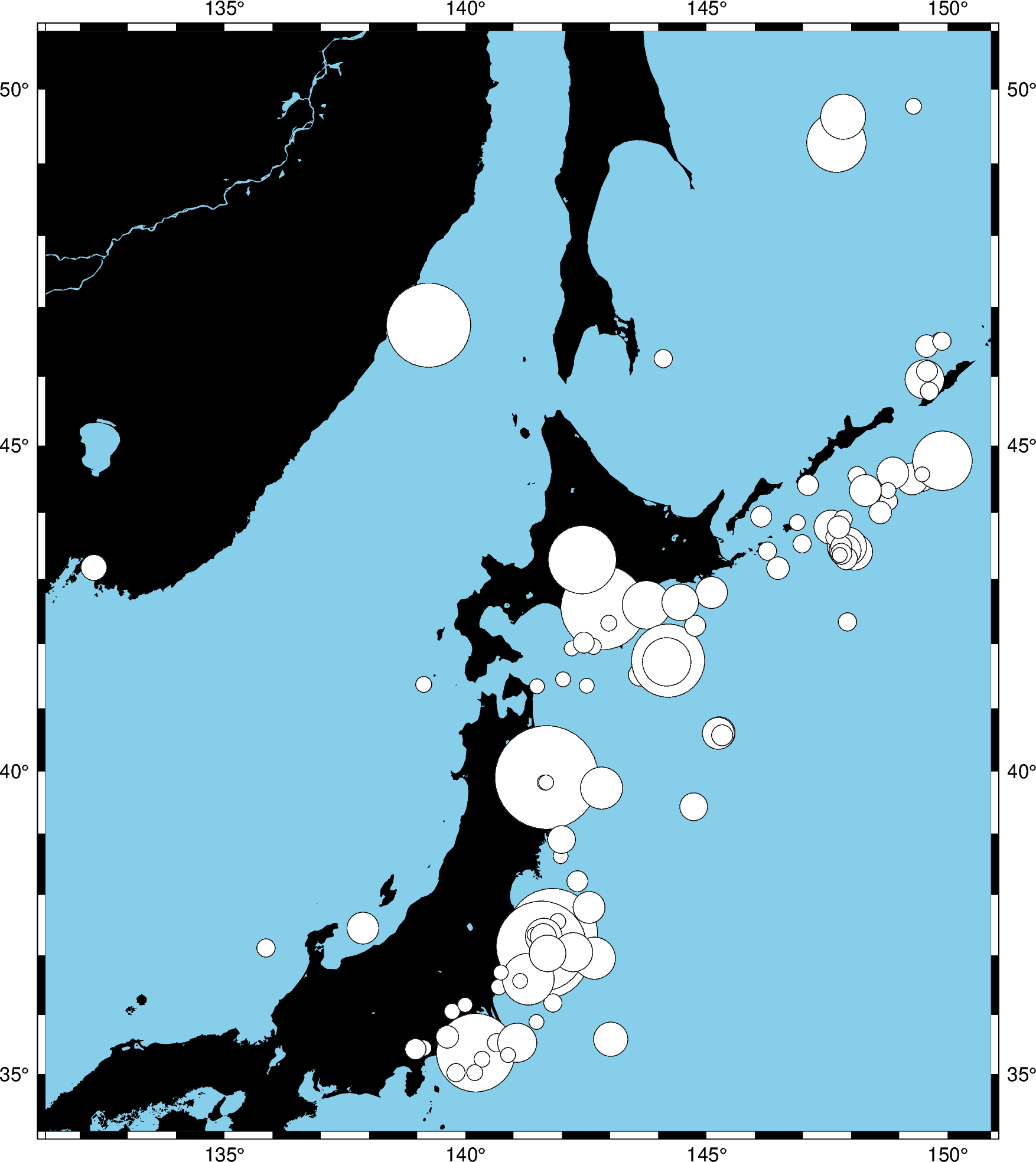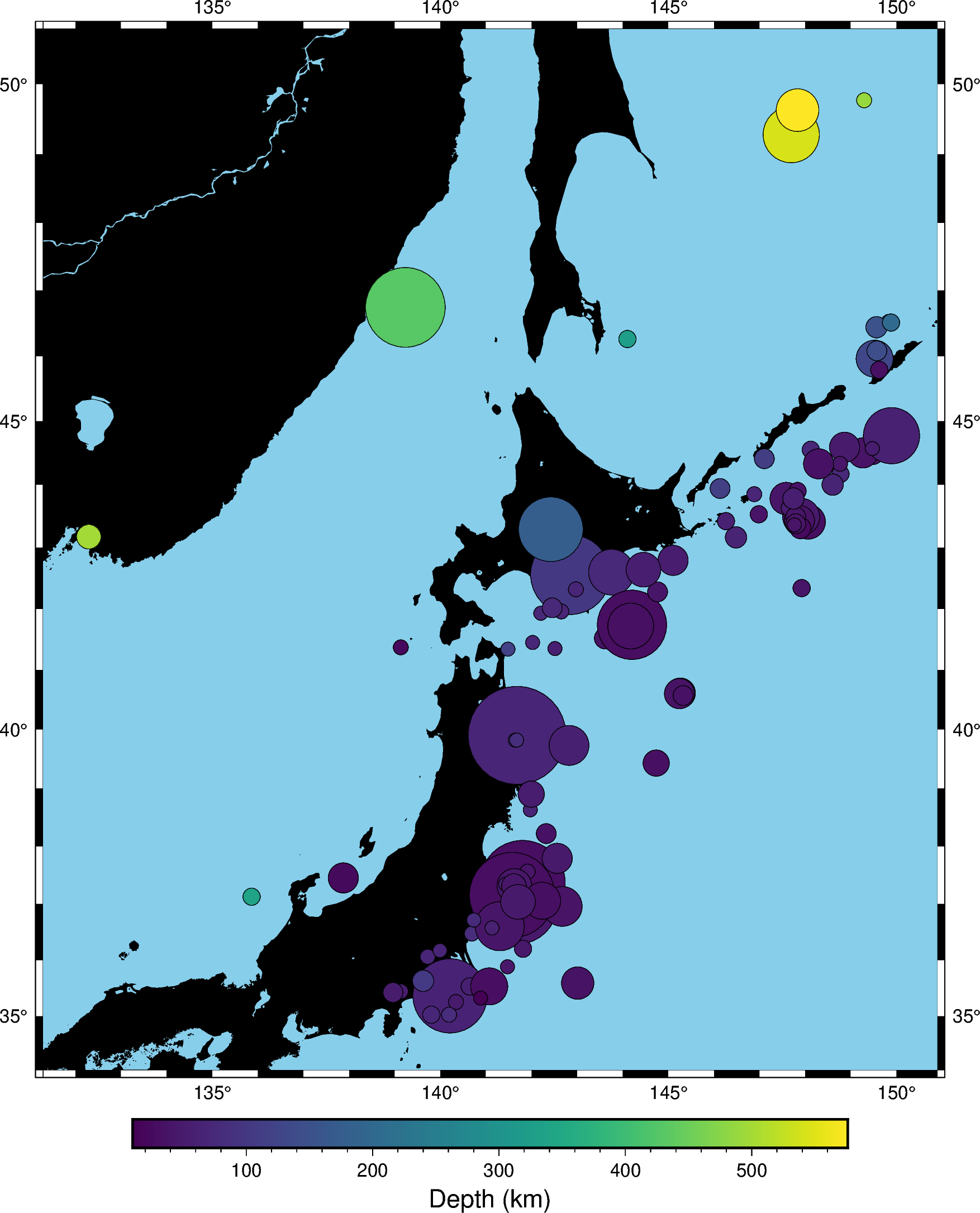# Plotting data points¶

GMT shines when it comes to plotting data on a map. We can use some sample data that is packaged with GMT to try this out. PyGMT provides access to these datasets through the pygmt.datasets package. If you don’t have the data files already, they are automatically downloaded and saved to a cache directory the first time you use them (usually ~/.gmt/cache).

import pygmt


For example, let’s load the sample dataset of tsunami generating earthquakes around Japan (pygmt.datasets.load_japan_quakes). The data is loaded as a pandas.DataFrame.

data = pygmt.datasets.load_japan_quakes()

# Set the region for the plot to be slightly larger than the data bounds.
region = [
data.longitude.min() - 1,
data.longitude.max() + 1,
data.latitude.min() - 1,
data.latitude.max() + 1,
]

print(region)


Out:

[131.29, 150.89, 34.02, 50.77]
year  month  day  latitude  longitude  depth_km  magnitude
0  1987      1    4     49.77     149.29       489        4.1
1  1987      1    9     39.90     141.68        67        6.8
2  1987      1    9     39.82     141.64        84        4.0
3  1987      1   14     42.56     142.85       102        6.5
4  1987      1   16     42.79     145.10        54        5.1


We’ll use pygmt.Figure.plot method to plot circles on the locations of the hypocenters of the earthquakes.

fig = pygmt.Figure()
fig.basemap(region=region, projection="M8i", frame=True)
fig.coast(land="black", water="skyblue")
fig.plot(x=data.longitude, y=data.latitude, style="c0.3c", color="white", pen="black")
fig.show()Out:

<IPython.core.display.Image object>


We used the style c0.3c which means “circles of 0.3 centimeter size”. The pen attribute controls the outline of the symbols and the color controls the fill.

We can map the size of the circles to the earthquake magnitude by passing an array to the sizes argument. Because the magnitude is on a logarithmic scale, it helps to show the differences by scaling the values using a power law.

fig = pygmt.Figure()
fig.basemap(region=region, projection="M8i", frame=True)
fig.coast(land="black", water="skyblue")
fig.plot(
x=data.longitude,
y=data.latitude,
sizes=0.02 * (2 ** data.magnitude),
style="cc",
color="white",
pen="black",
)
fig.show()Out:

<IPython.core.display.Image object>


Notice that we didn’t include the size in the style argument this time, just the symbol c (circles) and the unit c (centimeter). So in this case, the sizes will be interpreted as being in centimeters.

We can also map the colors of the markers to the depths by passing an array to the color argument and providing a colormap name (cmap). We can even use the new matplotlib colormap “viridis”. Here, we first create a continuous colormap ranging from the minimum depth to the maximum depth of the earthquakes using pygmt.makecpt, then set cmap=True in pygmt.Figure.plot to use the colormap. At the end of the plot, we also plot a colorbar showing the colormap used in the plot.

fig = pygmt.Figure()
fig.basemap(region=region, projection="M8i", frame=True)
fig.coast(land="black", water="skyblue")
pygmt.makecpt(cmap="viridis", series=[data.depth_km.min(), data.depth_km.max()])
fig.plot(
x=data.longitude,
y=data.latitude,
sizes=0.02 * 2 ** data.magnitude,
color=data.depth_km,
cmap=True,
style="cc",
pen="black",
)
fig.colorbar(frame='af+l"Depth (km)"')
fig.show()Out:

<IPython.core.display.Image object>


Total running time of the script: ( 0 minutes 3.549 seconds)

Gallery generated by Sphinx-Gallery